home | O'Reilly's CD bookshelfs | FreeBSD | Linux | Cisco | Cisco Exam

#Chapter 17troff Preprocessors## 17.2 eqn

eqn is a preprocessor designed to facilitate the typesetting of mathematical equations. Use neqn with nroff . eqn has the following command-line syntax:

```eqn [options
] [files
]```

The canonical reference for eqn is Typesetting Mathematics -- User's Guide , by L.L. Cherry and B.W. Kernighan, in UNIX Programmer's Manual, Tenth Edition , Volume 2, AT&T Bell Laboratories, M.D. McIlroy and A.G. Hume editors, Holt Rinehart & Winston, 1990. This paper may be downloaded from http://cm.bell-labs.com/cm/cs/doc/74/eqn.ps.gz .

### 17.2.1 Options

`-d` xy

Use x and y as start and stop delimiters; same as specifying the eqn directive ```delim ``` xy .

`-f` n

Change to font n ; same as the ```gfont ``` directive.

`-p` n

Reduce size of superscripts and subscripts by n points. If `-p` is not specified, the default reduction is 3 points.

`-s` n

Reduce the point size by n points; same as the ```gsize ``` directive.

`-T` dev

Format output to device dev . The default value comes from the TYPESETTER environment variable. Not available with neqn . (This option is not on Solaris eqn .)

### 17.2.2 eqn Macros

 ```.EQ ``` Start typesetting mathematics. ```.EN ``` End typesetting mathematics.

Use the checkeq command to check for unmatched macro pairs. (Not all systems have it, though.)

### 17.2.3 Mathematical Characters

The character sequences below are recognized and translated as shown:

Character Translation Character Translation
```>= ```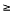```approx ```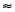```<= ```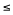```nothing ```
```== ```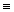```cdot ``` .
```!= ```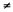```times ``` ×
```+- ``` ± ```del ```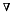```-> ```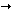```grad ``````<- ```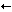```... ``` ...
```<< ```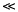```,..., ``` ,...,
```>> ```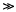```sum ```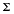```inf ```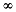```int ```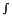```partial ```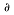```prod ```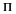```half ``` ½ ```union ```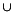```prime ```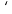```inter ```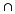### 17.2.4 Mathematical Text

Digits, parentheses, brackets, punctuation marks, and the following mathematical words are printed out in roman font:

 ```sin ``` ```cos ``` ```tan ``` ```arc ``` ```sinh ``` ```cosh ``` ```tanh ``` ```and ``` ```if ``` ```for ``` ```det ``` ```max ``` ```min ``` ```lim ``` ```log ``` ```ln ``` ```exp ``` ```Re ``` ```Im ```

### 17.2.5 Greek Characters

Greek letters can be printed in uppercase or lowercase. To obtain Greek letters, simply spell them out. Some uppercase Greek letters are not supported because they can be specified by a roman equivalent (e.g, A for alpha, B for beta).

Name Character Name Character
alpha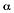tau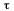beta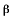upsilon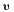gamma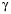phi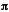delta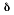chi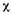epsilon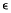psi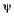zeta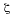omega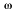eta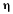GAMMA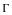theta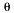DELTA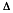iota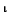THETA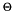kappa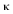LAMBDA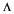lambda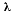XI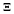mu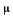PInu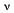SIGMAxi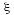UPSILON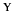omicron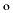PHI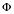piPSI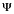rho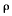OMEGA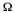sigma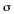### 17.2.6 Diacritical Marks

Several keywords are available to mark the tops of characters. eqn centers a mark at the correct height. ```bar ``` and ```under ``` span the necessary length.

Character Translation
```x dot ```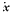```x dotdot ``````x hat ```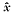```x tilde ```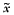```x vec ```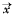```x dyad ```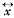```x bar ```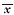```x under ```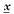### 17.2.7 Keywords Recognized by eqn

In addition to character names and diacritical marks, eqn recognizes the following keywords.

 ```above ``` Separate the pieces of a pile or matrix column. ```back ``` n Move backwards horizontally n 1/100s of an em. ```bold ``` Change to bold font. ```ccol ``` Center-align a column of a matrix. ```cpile ``` Make a centered pile (same as ```pile ``` ). ```define ``` Create a name for a frequently used string. ```delim ``` xy Define two characters to mark the left and right ends of an eqn equation to be printed inline. Use ```delim off ``` to turn off delimiters. ```down ``` n Move down n 1/100s of an em. ```fat ``` Widen the current font by overstriking it. ```font ``` x Change to font x , where x is the name or number of a font. ```from ``` Used in summations, integrals, and similar constructions to signify the lower limit. ```fwd ``` n Move forward horizontally n 1/100s of an em. ```gfont ``` x Set a global font x for all equations. ```gsize ``` n Set a global size for all equations. ```italic ``` Change to italic font. ```lcol ``` Left-justify a column of a matrix. ```left ``` Create big brackets, big braces, big bars, etc. ```lineup ``` Line up marks in equations on different lines. ```lpile ``` Left-justify the elements of a pile. ```mark ``` Remember the horizontal position in an equation. Used with ```lineup ``` . ```matrix ``` Create a matrix. ```ndefine ``` Create a definition that takes effect only when neqn is running. ```over ``` Make a fraction. ```pile ``` Make a vertical pile with elements centered above each other. ```rcol ``` Right-adjust a column of a matrix. ```right ``` Create big brackets, big braces, big bars, etc. Must have a matching ```left ``` . ```roman ``` Set following constant in roman. ```rpile ``` Right-justify the elements of a pile. ```size ``` n Change the size of the font to n . ```sqrt ``` Take the square root of the following equation element. ```sub ``` Start a subscript. ```sup ``` Start a superscript. ```tdefine ``` Make a definition that applies only to eqn . ```to ``` Used in summations, integrals, and similar constructions to signify the upper limit. ```up ``` n Move up n 1/100s of an em. ```~ ``` Force extra space into the output. ```^ ``` Force a space one-half the size of the space forced by ```~ ``` . ```{ } ``` Force eqn to treat an element as a unit. ```"..." ``` A string within quotes is not subject to alterations by eqn .

### 17.2.8 Precedence

If you don't use braces, eqn performs operations in the order shown in this list, reading from left to right.

 ```dyad ``` ```vec ``` ```under ``` ```bar ``` ```tilde ``` ```hat ``` ```dot ``` ```dotdot ``` ```fwd ``` ```back ``` ```down ``` ```up ``` ```fat ``` ```roman ``` ```italic ``` ```bold ``` ```size ``` ```sub ``` ```sup ``` ```sqrt ``` ```over ``` ```from ``` ```to ```

These operations group to the left:

 ```over ``` ```sqrt ``` ```left ``` ```right ```

All others group to the right.

eqn defines a language for writing mathematics. Thus, there is a grammar with rules about how to group and order items within the equation. See the Bell Labs memorandum for the full story.

### 17.2.9 eqn Examples

Input:

```.EQ
delim %%
.EN
%sum from i=0 to inf c sup i~=~lim from {m -> inf}
sum from i=0 to m c sup i%
.EQ
delim off
.EN```

Result: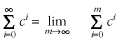Input:

```.EQ
x ~=~ left [ { -b ~+-~ sqrt {b sup 2 - ~4ac} }
over 2a right ]
.EN```

Result: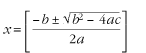17.1 tbl17.3 pic